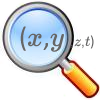# Resources tagged with: Trigonometric functions and graphs

Filter by: Content type:
Age range:
Challenge level:

### There are 14 results

Broad Topics > Coordinates, Functions and Graphs > Trigonometric functions and graphs### What Do Functions Do for Tiny X?

##### Age 16 to 18Challenge Level

Looking at small values of functions. Motivating the existence of the Taylor expansion.### Building Approximations for Sin(x)

##### Age 16 to 18Challenge Level

Build up the concept of the Taylor series### Taking Trigonometry Series-ly

##### Age 16 to 18Challenge Level

Look at the advanced way of viewing sin and cos through their power series.### Trig-trig

##### Age 16 to 18Challenge Level

Explore the properties of combinations of trig functions in this open investigation.### Trigger

##### Age 16 to 18 ShortChallenge Level

Can you sketch this tricky trig function?### Loch Ness

##### Age 16 to 18Challenge Level

Draw graphs of the sine and modulus functions and explain the humps.### Spherical Triangles on Very Big Spheres

##### Age 16 to 18Challenge Level

Shows that Pythagoras for Spherical Triangles reduces to Pythagoras's Theorem in the plane when the triangles are small relative to the radius of the sphere.### After Thought

##### Age 16 to 18Challenge Level

Which is larger cos(sin x) or sin(cos x) ? Does this depend on x ?### Small Steps

##### Age 16 to 18Challenge Level

Two problems about infinite processes where smaller and smaller steps are taken and you have to discover what happens in the limit.### Tangled Trig Graphs

##### Age 16 to 18Challenge Level

Can you work out the equations of the trig graphs I used to make my pattern?### Degree Ceremony

##### Age 16 to 18Challenge Level

What does Pythagoras' Theorem tell you about these angles: 90Â°, (45+x)Â° and (45-x)Â° in a triangle?### Climbing

##### Age 16 to 18Challenge Level

Sketch the graphs of y = sin x and y = tan x and some straight lines. Prove some inequalities.### Squareness

##### Age 16 to 18Challenge Level

The family of graphs of x^n + y^n =1 (for even n) includes the circle. Why do the graphs look more and more square as n increases?### Sine and Cosine

##### Age 14 to 16Challenge Level

The sine of an angle is equal to the cosine of its complement. Can you explain why and does this rule extend beyond angles of 90 degrees?